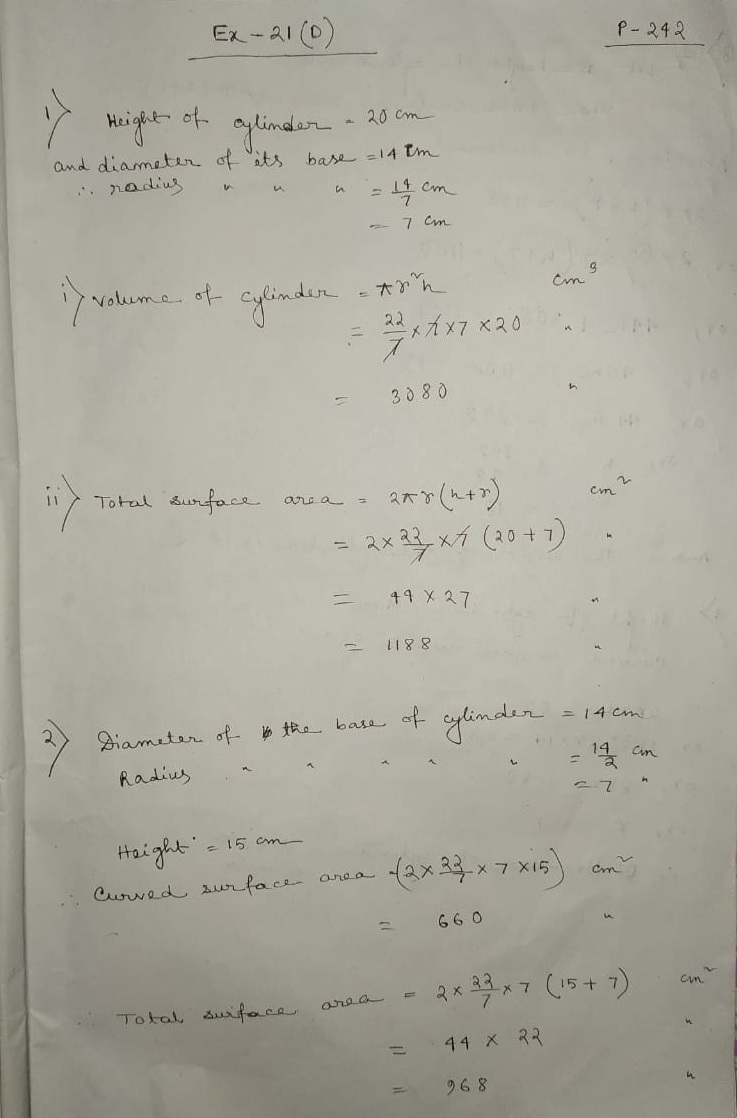# Selina Concise Class 8 Math Chapter 21 Surface Area Volume and Capacity Exercise 21D Solution

### Selina Concise Class 8 Math Chapter 21 Surface Area Volume and Capacity Exercise 21D Solution

Concise Mathematics – Class 8 Selina Exercise 21D Solution by experts | 21D Selina Concise Solution Class 8 | Class 8 Selina Concise Ex 21D Page 242 Solution | RK Bansal Class 8 ICSE Book Solution Online.

(1) The height of a cfircular cylinder is 20 cm and the diameter of its base is 14 cm. Find:
(i) its volume (ii) its total surface area.
(2) Find the curved surface area and the total surface area of a right circular cylinder whose height is 15 cm and the diameter of the cross section is 14 cm.

Solution:(3) Find the height of the cylinder whose radius is 7 cm and the total surface are is 1100 cm square.
(4) The curved surface area of a cylinder of height 14 cm is 88 cm square. Find the diameter of the base of the cylinder.

Solution:(5) The ration between the curved surface area and the total area of a cylinder is 1:2. Find the ration between the height and the radius of the cylinder.
(6) Find the capacity of a cylindrical container with internal diameter 28 cm and height 20 cm.

Solution:(7) The total surface area of a cylinder is 6512 cm square and the circumfarence of its base is 88 cm. Find:
(i) its radius (ii) its volume

Solution:(8) The sum of the radius and the height of a cylinder is 37 cm and the total surface area of the cylinder is 1628 cm square. Find the height and the volume of the cylinder.

(9) A cylindrical pillar has radius 21 cm and height 4 m. Find:
(i) the curved surface area of the pillar
(ii) cost of polishing 36 such cylindrical pillars at the rate of Rs. 12 per meter square.

Solution:(10) If radii of two cylinders are in the ratio 4:3 and their hights are in the ratio 5:6, find the ratio of their curved surfaces.

Solution:Updated: November 26, 2019 — 12:13 pm